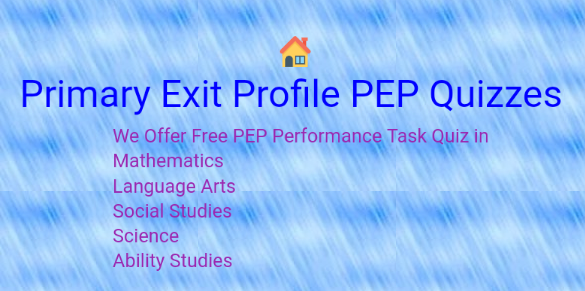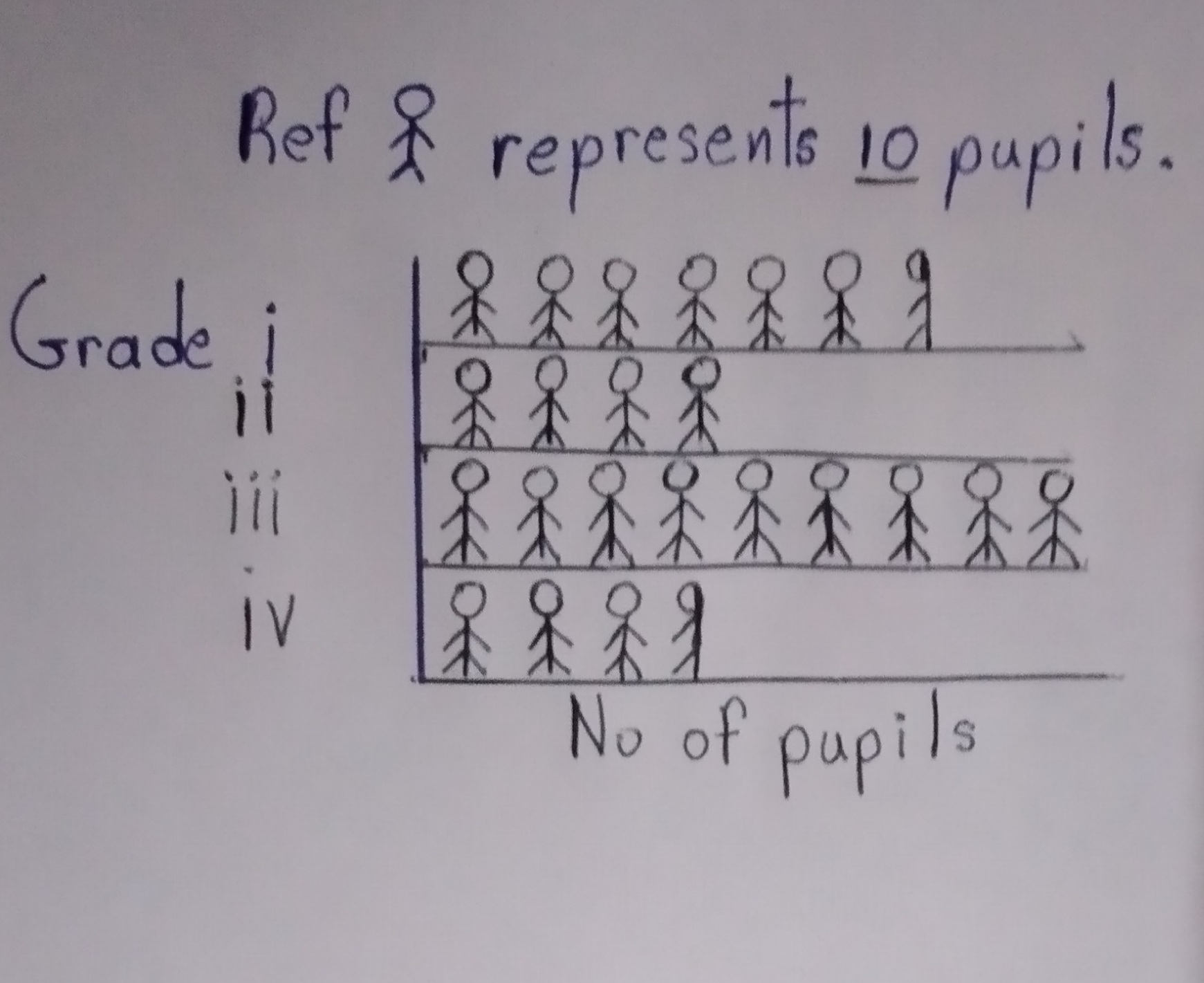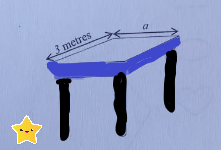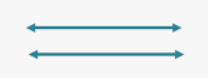Hardwork brings success, practice and you will become perfect. When finish share this app below using WhatsApp and Facebook.• Graphs are used to represent information in simple terms. Some of the graphs we will consider are:a) Pictographs b)Bar Graphs c) Line Graphs, d) Pie (circle) Graphs (Pie Charts)

Today lesson we will be looking at Pictographs.
As the word suggests, a pictograph is a picture graph. We use drawings or pictures to reprsent information. Study the pictograph below then answer the questions that follows.(1) Which grade has the most pupils?

(2)Which grade has the least number of pupils?(2 marks)

How many pupils are in grade 1?

(5) If 30 pupils form a class, how many classes has grade iii?

(6) Find the total number of pupils in the school.

(7) The perimeter of the top of a rectangle table is 10 metres.The length of one side of the table is 3 metres.What is the width of the side labelled a?(8) Write the name that best describe the lines above.

(9) Which angle on the diagram below is less than 90 degrees.PEP Mathematics Curriculum Based Test Grade 6 Quiz